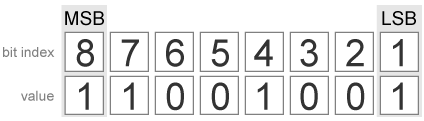# bitset

Set bit at specific location

## Syntax

``C = bitset(A,bit)``
``C = bitset(A,bit,V)``

## Description

example

````C = bitset(A,bit)` returns the value of `A` with position `bit` set to `1` (on).```

example

````C = bitset(A,bit,V)` returns the value of `A` with position `bit` set to `V`. ```

## Examples

collapse all

Begin with an unsigned fixed-point `fi` number with a value of 5, word length 4, and fraction length 0.

```a = fi(5,0,4,0); disp(bin(a))```
```0101 ```

Set the bit at position 4 to `1` (on).

```c = bitset(a,4); disp(bin(c))```
```1101 ```

Consider the following fixed-point vector with word length 4 and fraction length 0.

```a = fi([0 1 8 2 4],0,4,0); disp(bin(a))```
```0000 0001 1000 0010 0100 ```

In each element of vector `a`, set the bits at position 2 to `1`.

```c = bitset(a,2,1); disp(bin(c))```
```0010 0011 1010 0010 0110 ```

Consider the following fixed-point scalar with a value of 5.

```a = fi(5,0,4,0); disp(bin(a))```
```0101 ```

Set the bit at position `fi(2)` to `1`.

```c = bitset(a,fi(2),1); disp(bin(c))```
```0111 ```

Create a `fi` object with a value of `pi`.

```a = fi(pi); disp(bin(a))```
```0110010010001000 ```

In this case, `a` is signed with a word length of 16.

Create a vector of the bit positions in `a` that you want to set to on. Then, get the binary representation of the resulting `fi` vector.

```bit = fi([15,3,8,2]); c = bitset(a,bit); disp(bin(c))```
```0110010010001000 0110010010001100 0110010010001000 0110010010001010 ```

## Input Arguments

collapse all

Input values, specified as a scalar, vector, matrix, or multidimensional array of fixed-point `fi` objects. If any of `A`, `bit`, or `V` are nonscalar, the other inputs must be scalar or arrays of the same size. If `A` has a signed `numerictype`, the bit representation of the stored integer is in two's complement representation.

Data Types: `fi`
Complex Number Support: Yes

Bit position, specified as an integer or integer array of `fi` objects or built-in data types. If any of `A`, `bit`, or `V` are nonscalar, the other inputs must be scalar or arrays of the same size. The values of `bit` must be between `1` and the word length of `A`, inclusive. The `LSB`, the right-most bit, is specified by bit index `1`. The `MSB`, the left-most bit, is specified by the word length of `A`.

```a = fi(pi,0,8); a.bin```
```ans = '11001001'```Data Types: `single` | `double` | `int8` | `int16` | `int32` | `int64` | `uint8` | `uint16` | `uint32` | `uint64` | `fi`

Bit value of `A` at index `bit`, specified as a scalar, vector, matrix, or multidimensional array of `fi` objects or built-in data types. If any of `A`, `bit`, or `V` are nonscalar, the other inputs must be scalar or arrays of the same size. `V` can have values of `0` or `1`. Any value other than `0` is automatically set to `1`.

Data Types: `single` | `double` | `int8` | `int16` | `int32` | `int64` | `uint8` | `uint16` | `uint32` | `uint64` | `fi`
Complex Number Support: Yes

## Output Arguments

collapse all

Output array, specified as a scalar, vector, matrix, or multidimensional array of `fi` objects.

• If `A`, `bit`, and `V` are all scalars, then `C` is also a scalar.

• If any of `A`, `bit`, or `V` is an array, then `C` is the same size as that array.

## Version History

Introduced before R2006a

expand all